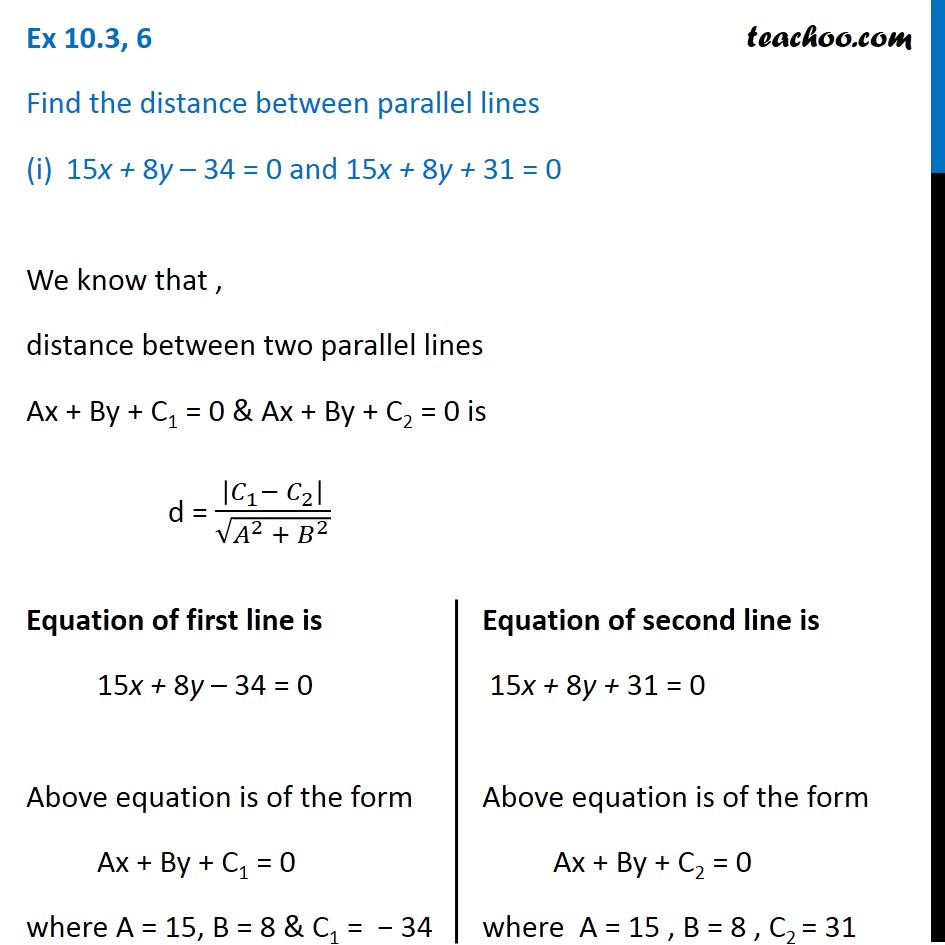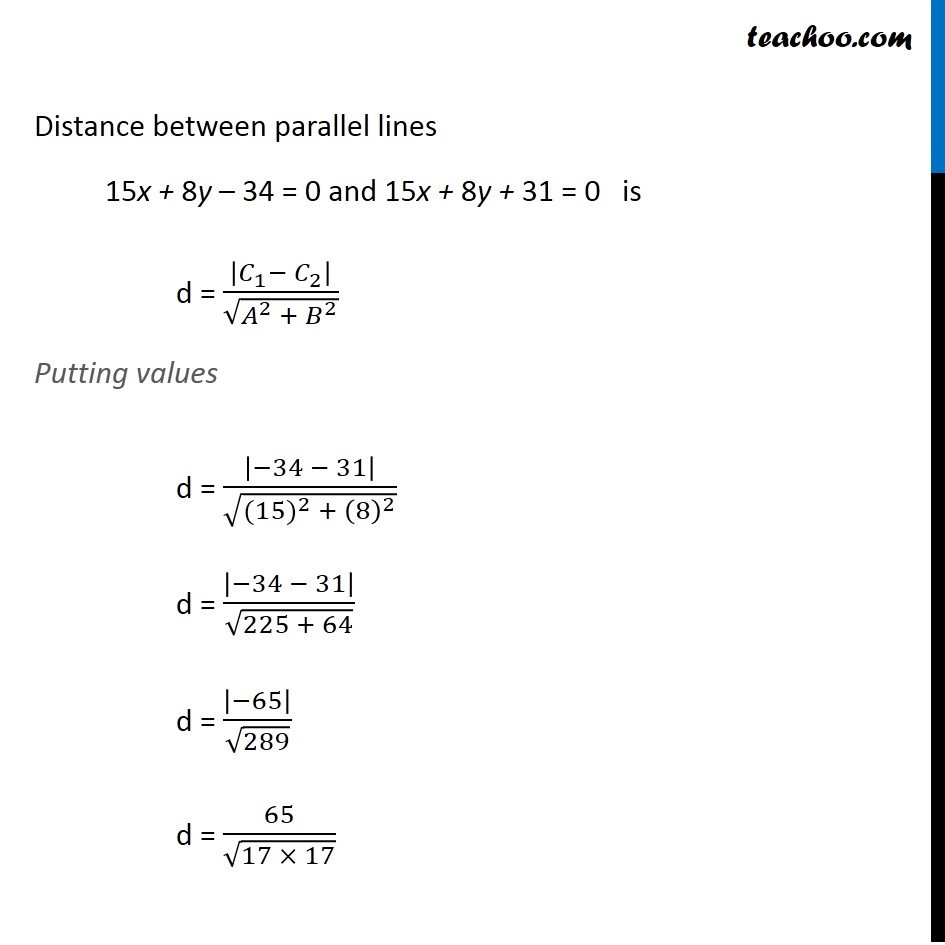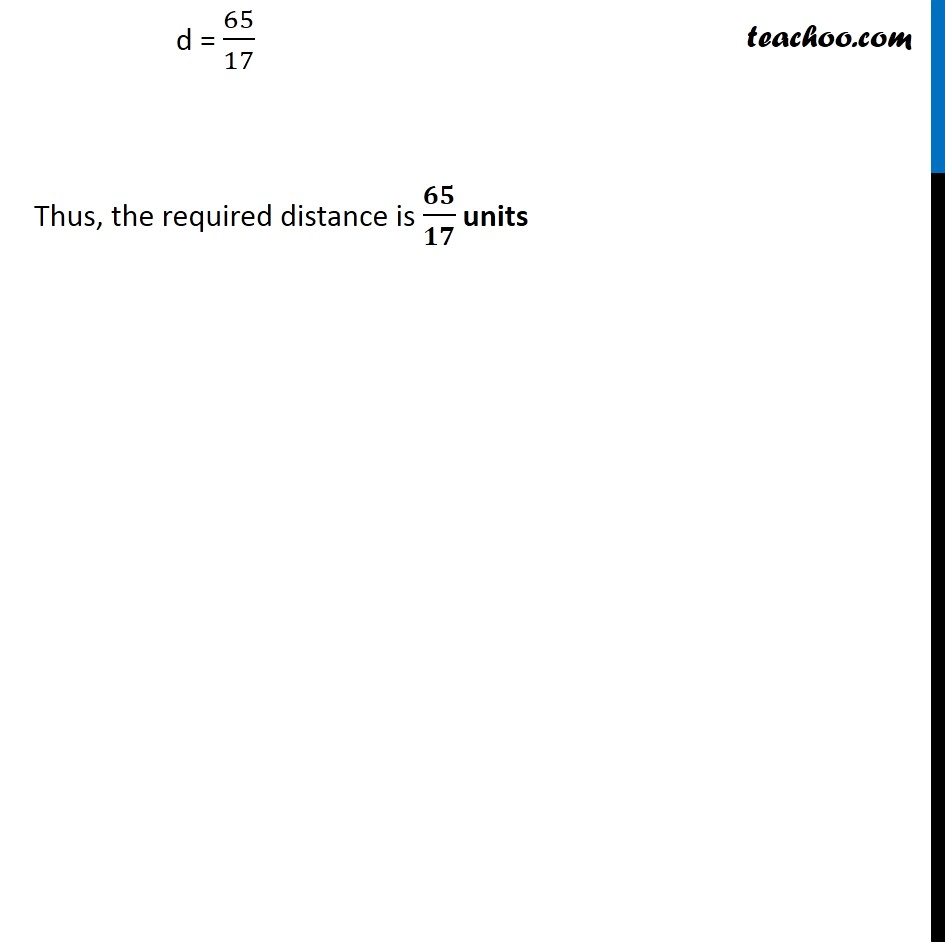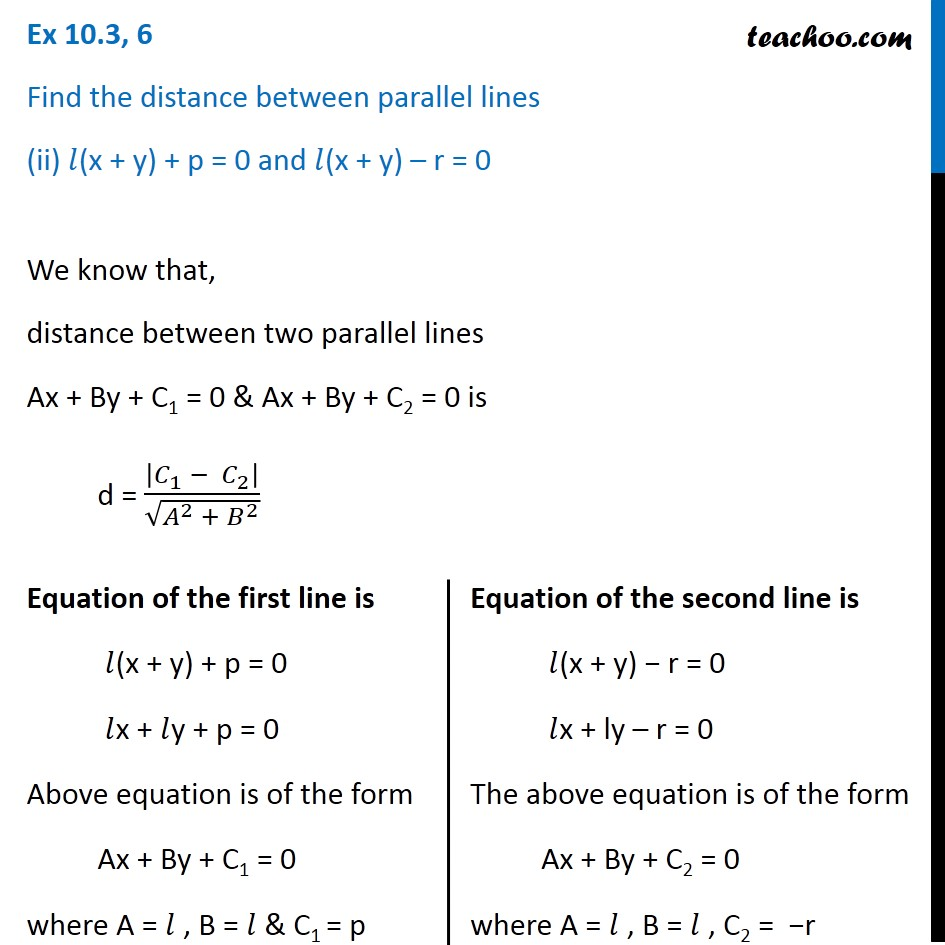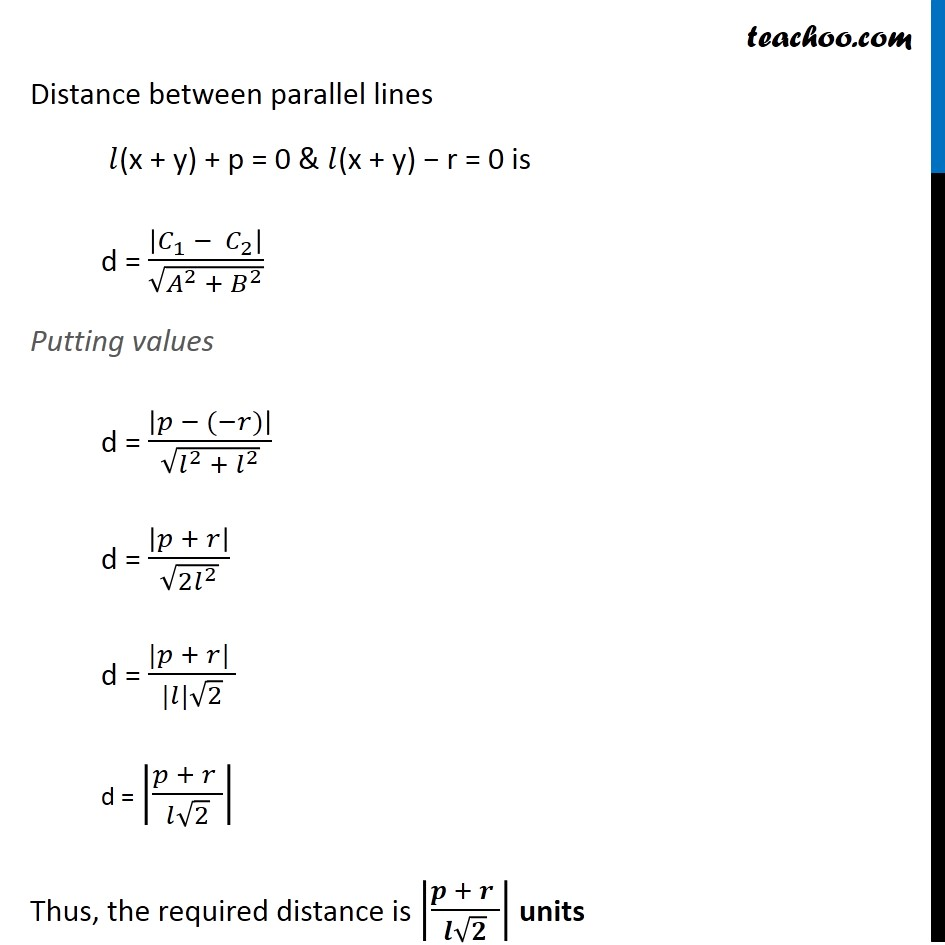Subscribe to our Youtube Channel - https://you.tube/teachoo

1. Chapter 10 Class 11 Straight Lines
2. Serial order wise
3. Ex 10.3

Transcript

Ex 10.3, 6 Find the distance between parallel lines 15x + 8y – 34 = 0 and 15x + 8y + 31 = 0 We know that , distance between two parallel lines Ax + By + C1 = 0 & Ax + By + C2 = 0 is d = |𝐶_1− 𝐶_2 |/√(𝐴^2 + 𝐵^2 ) Equation of first line is 15x + 8y – 34 = 0 Above equation is of the form Ax + By + C1 = 0 where A = 15, B = 8 & C1 = − 34 Equation of second line is 15x + 8y + 31 = 0 Above equation is of the form Ax + By + C2 = 0 where A = 15 , B = 8 , C2 = 31 Distance between parallel lines 15x + 8y – 34 = 0 and 15x + 8y + 31 = 0 is d = |𝐶_1− 𝐶_2 |/√(𝐴^2 + 𝐵^2 ) Putting values d = |−34 − 31|/√(〖(15)〗^2 + (8)^2 ) d = |−34 − 31|/√(225 + 64) d = |−65|/√289 d = 65/√(17 × 17) d = 65/17 Thus, the required distance is 𝟔𝟓/𝟏𝟕 units Ex 10.3, 6 Find the distance between parallel lines (ii) 𝑙(x + y) + p = 0 and 𝑙(x + y) – r = 0 We know that, distance between two parallel lines Ax + By + C1 = 0 & Ax + By + C2 = 0 is d = |𝐶_1 − 𝐶_2 |/√(𝐴^2 + 𝐵^2 ) Equation of the first line is 𝑙(x + y) + p = 0 𝑙x + 𝑙y + p = 0 Above equation is of the form Ax + By + C1 = 0 where A = 𝑙 , B = 𝑙 & C1 = p Equation of the second line is 𝑙(x + y) − r = 0 𝑙x + ly – r = 0 The above equation is of the form Ax + By + C2 = 0 where A = 𝑙 , B = 𝑙 , C2 = −r Distance between parallel lines 𝑙(x + y) + p = 0 & 𝑙(x + y) − r = 0 is d = |𝐶_1 − 𝐶_2 |/√(𝐴^2 + 𝐵^2 ) Putting values d = |𝑝 − (−𝑟)|/√(𝑙^2 + 𝑙^2 ) d = |𝑝 + 𝑟|/√(2𝑙^2 ) d = (|𝑝 + 𝑟| )/(|𝑙|√2) d = |(𝑝 + 𝑟 )/(𝑙√2)| Thus, the required distance is |(𝒑 + 𝒓 )/(𝒍√𝟐)| units

Ex 10.3